# Algorithm Introduction

8. Jun 2016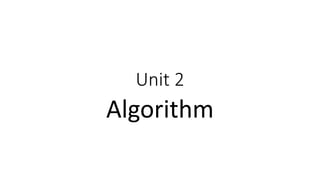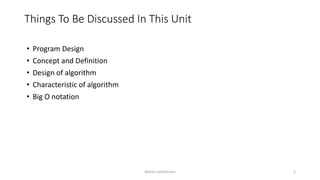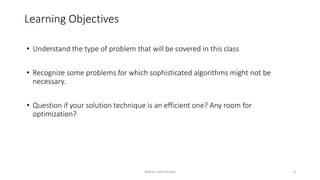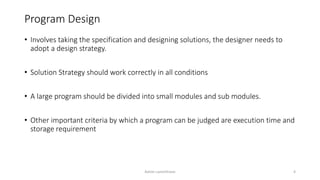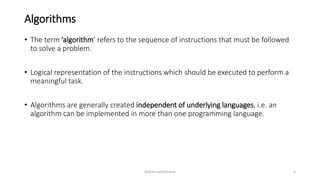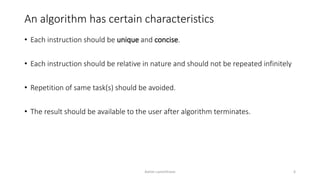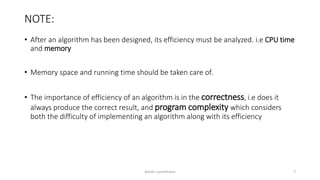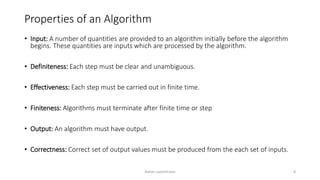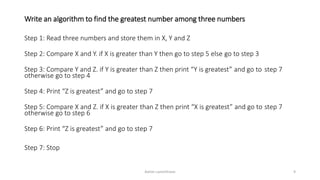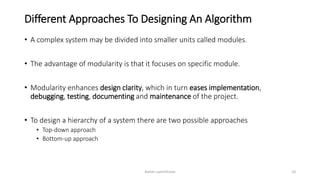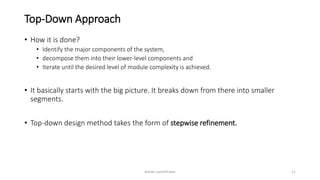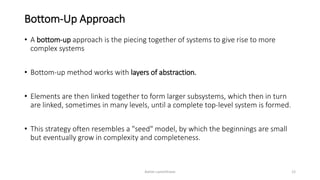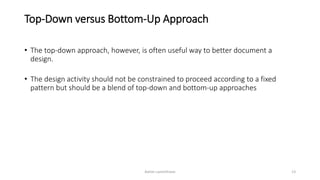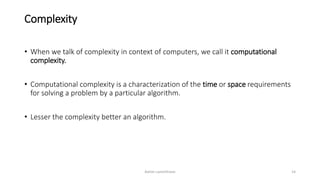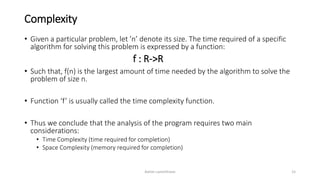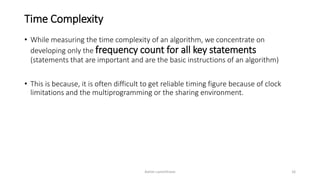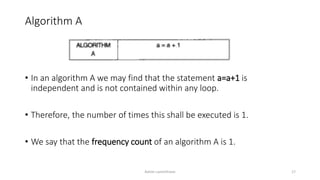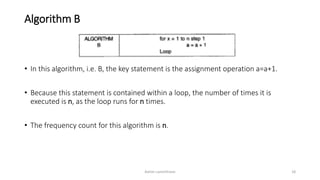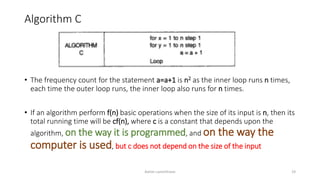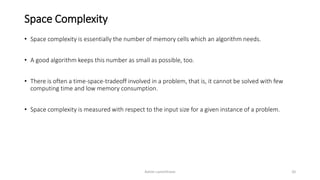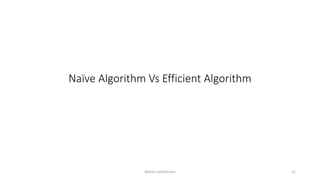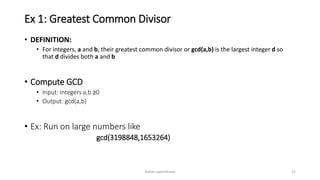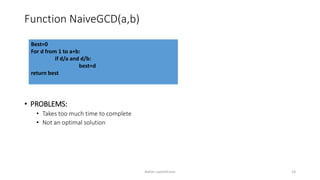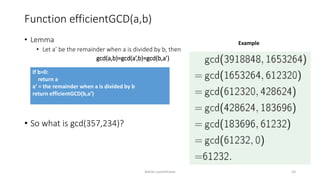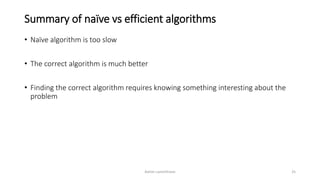1 von 26

### Algorithm Introduction

• 2. Things To Be Discussed In This Unit • Program Design • Concept and Definition • Design of algorithm • Characteristic of algorithm • Big O notation Ashim Lamichhane 2
• 3. Learning Objectives • Understand the type of problem that will be covered in this class • Recognize some problems for which sophisticated algorithms might not be necessary. • Question if your solution technique is an efficient one? Any room for optimization? Ashim Lamichhane 3
• 4. Program Design • Involves taking the specification and designing solutions, the designer needs to adopt a design strategy. • Solution Strategy should work correctly in all conditions • A large program should be divided into small modules and sub modules. • Other important criteria by which a program can be judged are execution time and storage requirement Ashim Lamichhane 4
• 5. Algorithms • The term ’algorithm’ refers to the sequence of instructions that must be followed to solve a problem. • Logical representation of the instructions which should be executed to perform a meaningful task. • Algorithms are generally created independent of underlying languages, i.e. an algorithm can be implemented in more than one programming language. Ashim Lamichhane 5
• 6. An algorithm has certain characteristics • Each instruction should be unique and concise. • Each instruction should be relative in nature and should not be repeated infinitely • Repetition of same task(s) should be avoided. • The result should be available to the user after algorithm terminates. Ashim Lamichhane 6
• 7. NOTE: • After an algorithm has been designed, its efficiency must be analyzed. i.e CPU time and memory • Memory space and running time should be taken care of. • The importance of efficiency of an algorithm is in the correctness, i.e does it always produce the correct result, and program complexity which considers both the difficulty of implementing an algorithm along with its efficiency Ashim Lamichhane 7
• 8. Properties of an Algorithm • Input: A number of quantities are provided to an algorithm initially before the algorithm begins. These quantities are inputs which are processed by the algorithm. • Definiteness: Each step must be clear and unambiguous. • Effectiveness: Each step must be carried out in finite time. • Finiteness: Algorithms must terminate after finite time or step • Output: An algorithm must have output. • Correctness: Correct set of output values must be produced from the each set of inputs. Ashim Lamichhane 8
• 9. Write an algorithm to find the greatest number among three numbers Step 1: Read three numbers and store them in X, Y and Z Step 2: Compare X and Y. if X is greater than Y then go to step 5 else go to step 3 Step 3: Compare Y and Z. if Y is greater than Z then print “Y is greatest” and go to step 7 otherwise go to step 4 Step 4: Print “Z is greatest” and go to step 7 Step 5: Compare X and Z. if X is greater than Z then print “X is greatest” and go to step 7 otherwise go to step 6 Step 6: Print “Z is greatest” and go to step 7 Step 7: Stop Ashim Lamichhane 9
• 10. Different Approaches To Designing An Algorithm • A complex system may be divided into smaller units called modules. • The advantage of modularity is that it focuses on specific module. • Modularity enhances design clarity, which in turn eases implementation, debugging, testing, documenting and maintenance of the project. • To design a hierarchy of a system there are two possible approaches • Top-down approach • Bottom-up approach Ashim Lamichhane 10
• 11. Top-Down Approach • How it is done? • Identify the major components of the system, • decompose them into their lower-level components and • Iterate until the desired level of module complexity is achieved. • It basically starts with the big picture. It breaks down from there into smaller segments. • Top-down design method takes the form of stepwise refinement. Ashim Lamichhane 11
• 12. Bottom-Up Approach • A bottom-up approach is the piecing together of systems to give rise to more complex systems • Bottom-up method works with layers of abstraction. • Elements are then linked together to form larger subsystems, which then in turn are linked, sometimes in many levels, until a complete top-level system is formed. • This strategy often resembles a "seed" model, by which the beginnings are small but eventually grow in complexity and completeness. Ashim Lamichhane 12
• 13. Top-Down versus Bottom-Up Approach • The top-down approach, however, is often useful way to better document a design. • The design activity should not be constrained to proceed according to a fixed pattern but should be a blend of top-down and bottom-up approaches Ashim Lamichhane 13
• 14. Complexity • When we talk of complexity in context of computers, we call it computational complexity. • Computational complexity is a characterization of the time or space requirements for solving a problem by a particular algorithm. • Lesser the complexity better an algorithm. Ashim Lamichhane 14
• 15. Complexity • Given a particular problem, let ’n’ denote its size. The time required of a specific algorithm for solving this problem is expressed by a function: f : R->R • Such that, f(n) is the largest amount of time needed by the algorithm to solve the problem of size n. • Function ‘f’ is usually called the time complexity function. • Thus we conclude that the analysis of the program requires two main considerations: • Time Complexity (time required for completion) • Space Complexity (memory required for completion) Ashim Lamichhane 15
• 16. Time Complexity • While measuring the time complexity of an algorithm, we concentrate on developing only the frequency count for all key statements (statements that are important and are the basic instructions of an algorithm) • This is because, it is often difficult to get reliable timing figure because of clock limitations and the multiprogramming or the sharing environment. Ashim Lamichhane 16
• 17. Algorithm A • In an algorithm A we may find that the statement a=a+1 is independent and is not contained within any loop. • Therefore, the number of times this shall be executed is 1. • We say that the frequency count of an algorithm A is 1. Ashim Lamichhane 17
• 18. Algorithm B • In this algorithm, i.e. B, the key statement is the assignment operation a=a+1. • Because this statement is contained within a loop, the number of times it is executed is n, as the loop runs for n times. • The frequency count for this algorithm is n. Ashim Lamichhane 18
• 19. Algorithm C • The frequency count for the statement a=a+1 is n2 as the inner loop runs n times, each time the outer loop runs, the inner loop also runs for n times. • If an algorithm perform f(n) basic operations when the size of its input is n, then its total running time will be cf(n), where c is a constant that depends upon the algorithm, on the way it is programmed, and on the way the computer is used, but c does not depend on the size of the input Ashim Lamichhane 19
• 20. Space Complexity • Space complexity is essentially the number of memory cells which an algorithm needs. • A good algorithm keeps this number as small as possible, too. • There is often a time-space-tradeoff involved in a problem, that is, it cannot be solved with few computing time and low memory consumption. • Space complexity is measured with respect to the input size for a given instance of a problem. Ashim Lamichhane 20
• 21. Naïve Algorithm Vs Efficient Algorithm Ashim Lamichhane 21
• 22. Ex 1: Greatest Common Divisor • DEFINITION: • For integers, a and b, their greatest common divisor or gcd(a,b) is the largest integer d so that d divides both a and b • Compute GCD • Input: Integers a,b ≥0 • Output: gcd(a,b) • Ex: Run on large numbers like gcd(3198848,1653264) Ashim Lamichhane 22
• 23. Function NaiveGCD(a,b) • PROBLEMS: • Takes too much time to complete • Not an optimal solution Ashim Lamichhane 23 Best=0 For d from 1 to a+b: if d/a and d/b: best=d return best
• 24. Function efficientGCD(a,b) • Lemma • Let a’ be the remainder when a is divided by b, then gcd(a,b)=gcd(a’,b)=gcd(b,a’) • So what is gcd(357,234)? Ashim Lamichhane 24 If b=0: return a a’ = the remainder when a is divided by b return efficientGCD(b,a’) Example
• 25. Summary of naïve vs efficient algorithms • Naïve algorithm is too slow • The correct algorithm is much better • Finding the correct algorithm requires knowing something interesting about the problem Ashim Lamichhane 25
• 26. Big O notation • To be continued on next slide… Ashim Lamichhane 26